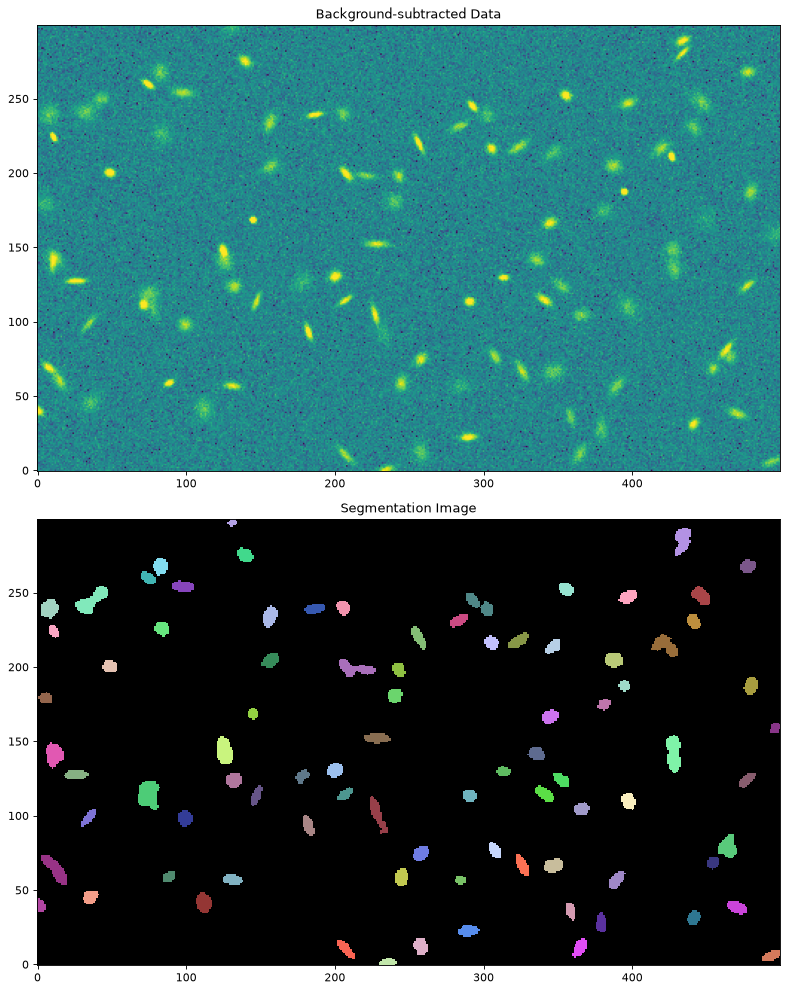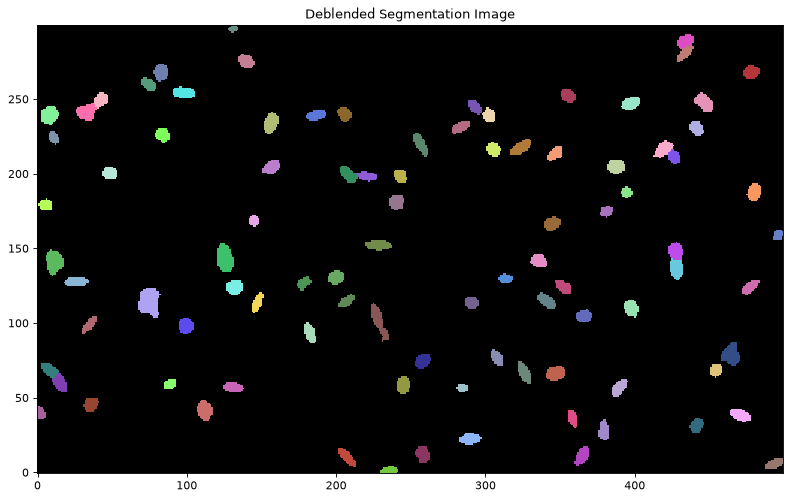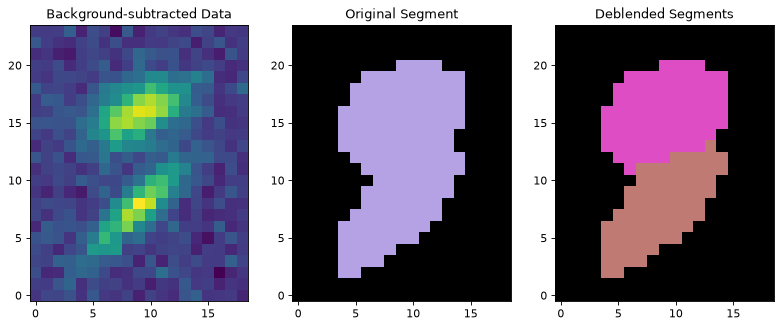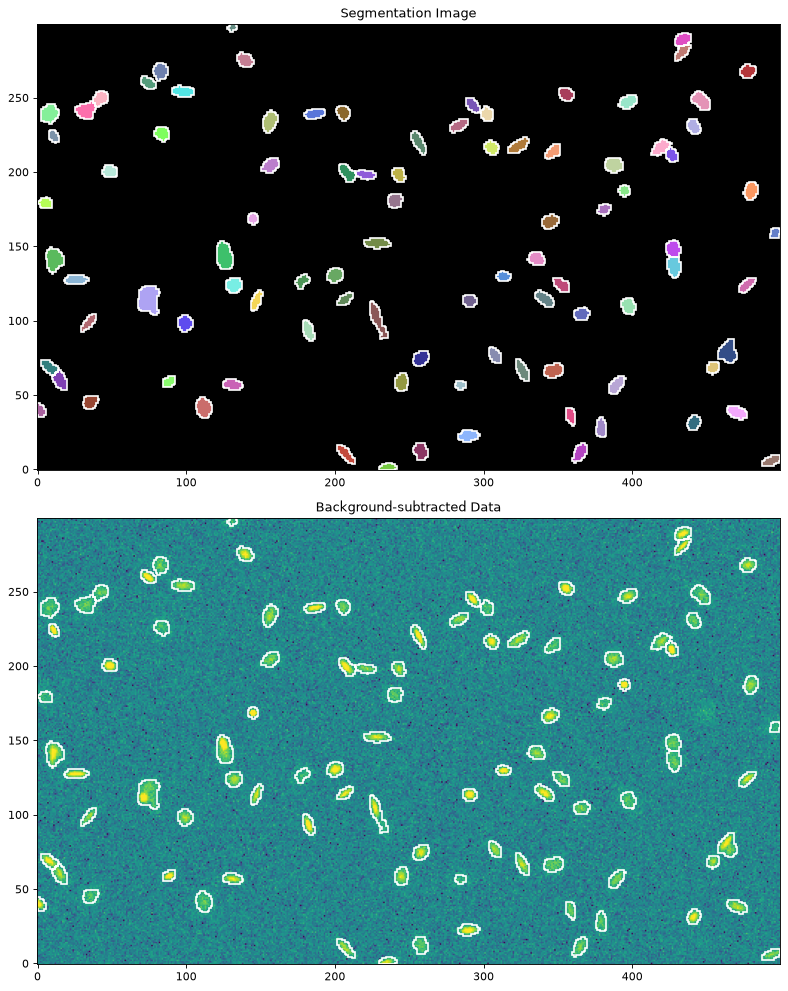# Image Segmentation (photutils.segmentation)¶

## Introduction¶

Photutils includes a general-use function to detect sources (both point-like and extended) in an image using a process called image segmentation in the computer vision field. After detecting sources using image segmentation, we can then measure their photometry, centroids, and morphological properties by using additional tools in Photutils.

## Source Extraction Using Image Segmentation¶

Photutils provides tools to detect astronomical sources using image segmentation, which is a process of assigning a label to every pixel in an image such that pixels with the same label are part of the same source. The segmentation procedure implemented in Photutils is called the threshold method, where detected sources must have a minimum number of connected pixels that are each greater than a specified threshold value in an image. The threshold level is usually defined at some multiple of the background noise (sigma) above the background. The image can also be filtered before thresholding to smooth the noise and maximize the detectability of objects with a shape similar to the filter kernel.

Let’s start by detecting sources in a synthetic image provided by the photutils.datasets module:

>>> from photutils.datasets import make_100gaussians_image
>>> data = make_100gaussians_image()


The source segmentation/extraction is performed using the detect_sources() function. We will use a convenience function called detect_threshold() to produce a 2D detection threshold image using simple sigma-clipped statistics to estimate the background level and RMS.

The threshold level is calculated using the nsigma input as the number of standard deviations (per pixel) above the background. Here we generate a simple threshold at 2 sigma (per pixel) above the background:

>>> from photutils import detect_threshold
>>> threshold = detect_threshold(data, nsigma=2.)


For more sophisticated analyses, one should generate a 2D background and background-only error image (e.g., from your data reduction or by using Background2D). In that case, a 2-sigma threshold image is simply:

>>> threshold = bkg + (2.0 * bkg_rms)


Note that if the threshold includes the background level (as above), then the image input into detect_sources() should not be background subtracted. In other words, the input threshold value(s) are compared directly to the input image. Because the threshold returned by detect_threshold() includes the background, we do not subtract the background from the data here.

Let’s find sources that have 5 connected pixels that are each greater than the corresponding pixel-wise threshold level defined above (i.e. 2 sigma per pixel above the background noise). Note that by default “connected pixels” means “8-connected” pixels, where pixels touch along their edges or corners. One can also use “4-connected” pixels that touch only along their edges by setting connectivity=4 in detect_sources().

We will also input a 2D circular Gaussian kernel with a FWHM of 3 pixels to smooth the image some prior to thresholding:

>>> from astropy.convolution import Gaussian2DKernel
>>> from astropy.stats import gaussian_fwhm_to_sigma
>>> from photutils import detect_sources
>>> sigma = 3.0 * gaussian_fwhm_to_sigma  # FWHM = 3.
>>> kernel = Gaussian2DKernel(sigma, x_size=3, y_size=3)
>>> kernel.normalize()
>>> segm = detect_sources(data, threshold, npixels=5, filter_kernel=kernel)


The result is a SegmentationImage object with the same shape as the data, where detected sources are labeled by different positive integer values. A value of zero is always reserved for the background. Let’s plot both the image and the segmentation image showing the detected sources:

>>> import numpy as np
>>> import matplotlib.pyplot as plt
>>> from astropy.visualization import SqrtStretch
>>> from astropy.visualization.mpl_normalize import ImageNormalize
>>> norm = ImageNormalize(stretch=SqrtStretch())
>>> fig, (ax1, ax2) = plt.subplots(2, 1, figsize=(10, 12.5))
>>> ax1.imshow(data, origin='lower', cmap='Greys_r', norm=norm)
>>> ax1.set_title('Data')
>>> cmap = segm.make_cmap(random_state=12345)
>>> ax2.imshow(segm, origin='lower', cmap=cmap)
>>> ax2.set_title('Segmentation Image')When the segmentation image is generated using image thresholding (e.g., using detect_sources()), the source segments represent the isophotal footprint of each source.

## Source Deblending¶

In the example above, overlapping sources are detected as single sources. Separating those sources requires a deblending procedure, such as a multi-thresholding technique used by SExtractor. Photutils provides a deblend_sources() function that deblends sources uses a combination of multi-thresholding and watershed segmentation. Note that in order to deblend sources, they must be separated enough such that there is a saddle between them.

The amount of deblending can be controlled with the two deblend_sources() keywords nlevels and contrast. nlevels is the number of multi-thresholding levels to use. contrast is the fraction of the total source flux that a local peak must have to be considered as a separate object.

Here’s a simple example of source deblending:

>>> from photutils import deblend_sources
>>> segm_deblend = deblend_sources(data, segm, npixels=5,
...                                filter_kernel=kernel, nlevels=32,
...                                contrast=0.001)


where segm is the SegmentationImage that was generated by detect_sources(). Note that the npixels and filter_kernel input values should match those used in detect_sources() to generate segm. The result is a new SegmentationImage object containing the deblended segmentation image:Let’s plot one of the deblended sources:## Modifying a Segmentation Image¶

The SegmentationImage object provides several methods that can be used to visualize or modify itself (e.g., combining labels, removing labels, removing border segments) prior to measuring source photometry and other source properties, including:

## Centroids, Photometry, and Morphological Properties¶

The source_properties() function is the primary tool for measuring the centroids, photometry, and morphological properties of sources defined in a segmentation image. When the segmentation image is generated using image thresholding (e.g., using detect_sources()), the source segments represent the isophotal footprint of each source and the resulting photometry is effectively isophotal photometry.

source_properties() returns a SourceCatalog object, which acts in part like a list of SourceProperties objects, one for each segmented source (or a specified subset of sources). An Astropy QTable of source properties can be generated using the to_table() method. Please see SourceProperties for the list of the many properties that are calculated for each source. More properties are likely to be added in the future.

Let’s detect sources and measure their properties in a synthetic image. For this example, we will use the Background2D class to produce a background and background noise image. We define a 2D detection threshold image using the background and background RMS images. We set the threshold at 2 sigma (per pixel) above the background:

>>> from astropy.convolution import Gaussian2DKernel
>>> from photutils.datasets import make_100gaussians_image
>>> from photutils import Background2D, MedianBackground
>>> from photutils import detect_threshold, detect_sources
>>> data = make_100gaussians_image()
>>> bkg_estimator = MedianBackground()
>>> bkg = Background2D(data, (50, 50), filter_size=(3, 3),
...                    bkg_estimator=bkg_estimator)
>>> threshold = bkg.background + (2. * bkg.background_rms)


Now we find sources that have 5 connected pixels that are each greater than the corresponding threshold image defined above. Because the threshold includes the background, we do not subtract the background from the data here. We also input a 2D circular Gaussian kernel with a FWHM of 3 pixels to filter the image prior to thresholding:

>>> from astropy.stats import gaussian_fwhm_to_sigma
>>> sigma = 3.0 * gaussian_fwhm_to_sigma  # FWHM = 3.
>>> kernel = Gaussian2DKernel(sigma, x_size=3, y_size=3)
>>> kernel.normalize()
>>> npixels = 5
>>> segm = detect_sources(data, threshold, npixels=npixels,
...                       filter_kernel=kernel)
>>> segm_deblend = deblend_sources(data, segm, npixels=npixels,
...                                filter_kernel=kernel, nlevels=32,
...                                contrast=0.001)


As described earlier, the result is a SegmentationImage where sources are labeled by different positive integer values.

Now let’s measure the properties of the detected sources defined in the segmentation image using the simplest call to source_properties(). The output QTable of source properties is generated by the SourceCatalog to_table() method. Each row in the table represents a source. The columns represent the calculated source properties. Note that the only a subset of the source properties are shown below. Please see SourceProperties for the list of the many properties that are calculated for each source:

>>> from photutils import source_properties
>>> cat = source_properties(data, segm_deblend)
>>> tbl = cat.to_table()
>>> tbl['xcentroid'].info.format = '.2f'  # optional format
>>> tbl['ycentroid'].info.format = '.2f'
>>> tbl['cxx'].info.format = '.2f'
>>> tbl['cxy'].info.format = '.2f'
>>> tbl['cyy'].info.format = '.2f'
>>> tbl['gini'].info.format = '.2f'
>>> print(tbl)
id xcentroid ycentroid sky_centroid ...   cxx      cxy      cyy    gini
pix       pix                 ... 1 / pix2 1 / pix2 1 / pix2
--- --------- --------- ------------ ... -------- -------- -------- ----
1    235.22      1.25         None ...     0.17    -0.20     0.99 0.18
2    493.82      5.77         None ...     0.16    -0.32     0.61 0.13
3    207.30     10.02         None ...     0.37     0.49     0.30 0.16
4    364.75     11.13         None ...     0.39    -0.33     0.18 0.13
5    258.37     11.77         None ...     0.37     0.15     0.16 0.13
...       ...       ...          ... ...      ...      ...      ...  ...
92    427.01    147.45         None ...     0.26    -0.07     0.12 0.12
93    426.60    211.14         None ...     0.67     0.24     0.35 0.41
94    419.79    216.68         None ...     0.17    -0.19     0.27 0.14
95    433.91    280.73         None ...     0.52    -0.83     0.49 0.23
96    434.11    288.90         None ...     0.18    -0.19     0.30 0.24
Length = 96 rows


Let’s use the measured morphological properties to define approximate isophotal ellipses for each source. Here we define an EllipticalAperture object for each source using its calculated centroid positions (xcentroid and ycentroid) , semimajor and semiminor axes lengths (semimajor_axis_sigma and semiminor_axis_sigma) , and orientation (orientation):

>>> import numpy as np
>>> import astropy.units as u
>>> from photutils import source_properties, EllipticalAperture
>>> cat = source_properties(data, segm_deblend)
>>> r = 3.  # approximate isophotal extent
>>> apertures = []
>>> for obj in cat:
...     position = np.transpose((obj.xcentroid.value, obj.ycentroid.value))
...     a = obj.semimajor_axis_sigma.value * r
...     b = obj.semiminor_axis_sigma.value * r
...     apertures.append(EllipticalAperture(position, a, b, theta=theta))


Now let’s plot the derived elliptical apertures on the data:

>>> import numpy as np
>>> import matplotlib.pyplot as plt
>>> from astropy.visualization import SqrtStretch
>>> from astropy.visualization.mpl_normalize import ImageNormalize
>>> norm = ImageNormalize(stretch=SqrtStretch())
>>> fig, (ax1, ax2) = plt.subplots(2, 1, figsize=(10, 12.5))
>>> ax1.imshow(data, origin='lower', cmap='Greys_r', norm=norm)
>>> ax1.set_title('Data')
>>> cmap = segm_deblend.make_cmap(random_state=12345)
>>> ax2.imshow(segm_deblend, origin='lower', cmap=cmap)
>>> ax2.set_title('Segmentation Image')
>>> for aperture in apertures:
...     aperture.plot(axes=ax1, color='white', lw=1.5)
...     aperture.plot(axes=ax2, color='white', lw=1.5)We can also specify a specific subset of sources, defined by their label numbers in the segmentation image:

>>> labels = [1, 5, 20, 50, 75, 80]
>>> cat = source_properties(data, segm_deblend, labels=labels)
>>> tbl2 = cat.to_table()
>>> tbl2['xcentroid'].info.format = '.2f'  # optional format
>>> tbl2['ycentroid'].info.format = '.2f'
>>> tbl2['cxx'].info.format = '.2f'
>>> tbl2['cxy'].info.format = '.2f'
>>> tbl2['cyy'].info.format = '.2f'
>>> tbl2['gini'].info.format = '.2f'
>>> print(tbl2)
id xcentroid ycentroid sky_centroid ...   cxx      cxy      cyy    gini
pix       pix                 ... 1 / pix2 1 / pix2 1 / pix2
--- --------- --------- ------------ ... -------- -------- -------- ----
1    235.22      1.25         None ...     0.17    -0.20     0.99 0.18
5    258.37     11.77         None ...     0.37     0.15     0.16 0.13
20    347.00     66.94         None ...     0.15    -0.01     0.21 0.11
50    145.06    168.55         None ...     0.66     0.05     0.71 0.45
75    301.86    239.25         None ...     0.47    -0.05     0.28 0.08
80     43.20    250.01         None ...     0.18    -0.08     0.34 0.11


By default, the to_table() method will include most scalar-valued properties from SourceProperties, but a subset of properties can also be specified (or excluded) in the QTable via the columns or exclude_columns keywords:

>>> labels = [1, 5, 20, 50, 75, 80]
>>> cat = source_properties(data, segm_deblend, labels=labels)
>>> columns = ['id', 'xcentroid', 'ycentroid', 'source_sum', 'area']
>>> tbl3 = cat.to_table(columns=columns)
>>> tbl3['xcentroid'].info.format = '.4f'  # optional format
>>> tbl3['ycentroid'].info.format = '.4f'
>>> tbl3['source_sum'].info.format = '.4f'
>>> print(tbl3)
id xcentroid ycentroid source_sum area
pix       pix               pix2
--- --------- --------- ---------- ----
1  235.2160    1.2457   594.2193 36.0
5  258.3710   11.7694   684.7155 58.0
20  346.9998   66.9428   864.9778 73.0
50  145.0591  168.5496   885.9582 33.0
75  301.8641  239.2534   391.1656 36.0
80   43.2023  250.0100   627.6727 55.0


A WCS transformation can also be input to source_properties() via the wcs keyword, in which case the sky coordinates at the source centroids will be returned.

### Background Properties¶

Like with aperture_photometry(), the data array that is input to source_properties() should be background subtracted. If you input the background image that was subtracted from the data into the background keyword of source_properties(), the background properties for each source will also be calculated:

>>> labels = [1, 5, 20, 50, 75, 80]
>>> cat = source_properties(data, segm_deblend, labels=labels,
...                         background=bkg.background)
>>> columns = ['id', 'background_at_centroid', 'background_mean',
...            'background_sum']
>>> tbl4 = cat.to_table(columns=columns)
>>> tbl4['background_at_centroid'].info.format = '{:.10f}'  # optional format
>>> tbl4['background_mean'].info.format = '{:.10f}'
>>> tbl4['background_sum'].info.format = '{:.10f}'
>>> print(tbl4)
id background_at_centroid background_mean background_sum
--- ---------------------- --------------- --------------
1           5.2020428266    5.2021662094 187.2779835383
5           5.2140031370    5.2139893924 302.4113847608
20           5.2787968578    5.2785772173 385.3361368595
50           5.1896511123    5.1895516008 171.2552028270
75           5.1409531509    5.1408425626 185.0703322539
80           5.2109780136    5.2108402505 286.5962137759


### Photometric Errors¶

source_properties() requires inputting a total error array, i.e. the background-only error plus Poisson noise due to individual sources. The calc_total_error() function can be used to calculate the total error array from a background-only error array and an effective gain.

The effective_gain, which is the ratio of counts (electrons or photons) to the units of the data, is used to include the Poisson noise from the sources. effective_gain can either be a scalar value or a 2D image with the same shape as the data. A 2D effective gain image is useful for mosaic images that have variable depths (i.e., exposure times) across the field. For example, one should use an exposure-time map as the effective_gain for a variable depth mosaic image in count-rate units.

Let’s assume our synthetic data is in units of electrons per second. In that case, the effective_gain should be the exposure time (here we set it to 500 seconds). Here we use calc_total_error() to calculate the total error and input it into the source_properties() function. When a total error is input, the source_sum_err property is calculated. source_sum and source_sum_err are the instrumental flux and propagated flux error within the source segments:

>>> from photutils.utils import calc_total_error
>>> labels = [1, 5, 20, 50, 75, 80]
>>> effective_gain = 500.
>>> error = calc_total_error(data, bkg.background_rms, effective_gain)
>>> cat = source_properties(data, segm_deblend, labels=labels, error=error)
>>> columns = ['id', 'xcentroid', 'ycentroid', 'source_sum',
...            'source_sum_err']
>>> tbl5 = cat.to_table(columns=columns)
>>> tbl5['xcentroid'].info.format = '{:.4f}'  # optional format
>>> tbl5['ycentroid'].info.format = '{:.4f}'
>>> for col in tbl5.colnames:
...     tbl5[col].info.format = '%.8g'  # for consistent table output
>>> print(tbl5)
id xcentroid ycentroid source_sum source_sum_err
pix       pix
--- --------- --------- ---------- --------------
1 235.21604 1.2457344  594.21933      12.787658
5 258.37099 11.769376  684.71547      16.326605
20 346.99975 66.942777  864.97776      18.677809
50 145.05911 168.54961   885.9582      11.908449
75 301.86414 239.25337  391.16559      12.080326
80 43.202278 250.00997  627.67268      15.812197


Pixels can be completely ignored/excluded (e.g. bad pixels) when measuring the source properties by providing a boolean mask image via the mask keyword (True pixel values are masked) to the source_properties() function or SourceProperties class.

### Filtering¶

SExtractor’s centroid and morphological parameters are always calculated from a filtered “detection” image. The usual downside of the filtering is the sources will be made more circular than they actually are (assuming a circular kernel is used, which is common). If you wish to reproduce SExtractor results, then use the source_properties() filter_kernel keyword to filter the data prior to centroid and morphological measurements. The kernel should be the same one used with detect_sources() to define the segmentation image. If filter_kernel is None, then the centroid and morphological measurements will be performed on the unfiltered data. Note that photometry is always performed on the unfiltered data.

## Reference/API¶

This subpackage contains tools for detecting sources using image segmentation and measuring their centroids, photometry, and morphological properties.

### Functions¶

 deblend_sources(data, segment_img, npixels) Deblend overlapping sources labeled in a segmentation image. detect_sources(data, threshold, npixels[, …]) Detect sources above a specified threshold value in an image and return a SegmentationImage object. make_source_mask(data, nsigma, npixels[, …]) Make a source mask using source segmentation and binary dilation. source_properties(data, segment_img[, …]) Calculate photometry and morphological properties of sources defined by a labeled segmentation image.

### Classes¶

 Segment(segment_data, label, slices, area) Class for a single labeled region (segment) within a segmentation image. Class for a segmentation image. SourceCatalog(properties_list[, wcs]) Class to hold source catalogs. SourceProperties(data, segment_img, label[, …]) Class to calculate photometry and morphological properties of a single labeled source.